# Grade 5 Data and Graphing Worksheets

## Introducing statistics

Our  grade 5 data and graphing exercises extend previous graphing skills (double bar and line graphs, line plots and circle graphs with fractions) and introduce basic probability and statistics (range, mode, mean, median).

 /*-->*/ Double bar graphs Create & analyze double bar graphs.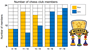/*-->*/ Double line graphs Create & analyze double line graphs.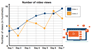/*-->*/ Line plots with fractions Make line plots with fractional values.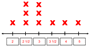Stem and leaf plots Create, read and analyze stem & leaf plots.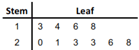/*-->*/ Circle graphs Interpret circle graphs using fractions.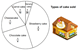/*-->*/ Probability Calculate simple probabilities as fractions.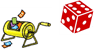/*-->*/ Range & mode Determine the range and mode of simple data sets.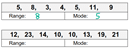/*-->*/ Range, median & mode Find the range, median & mode of simple data sets.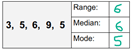/*-->*/ Mean Calculate the mean (average) of a data set.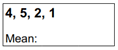Mean, median and mode Describe a data set by its mean, median and mode.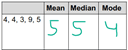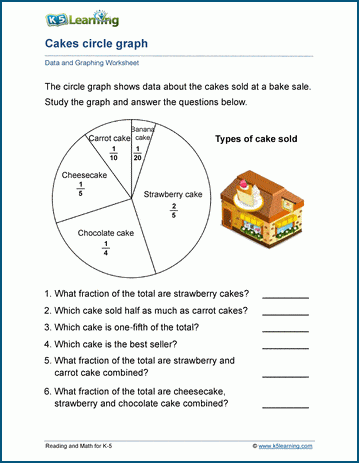Grade 5 data and graphing worksheet

What is K5?

K5 Learning offers free worksheets, flashcards and inexpensive workbooks for kids in kindergarten to grade 5. Become a member to access additional content and skip ads.